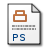# When maximising entropy gives the rational closure

Hill, L.C. and Paris, J.B. (2003) When maximising entropy gives the rational closure. Journal of Logic and Computation, 13 (1). pp. 51-68. ISSN 0955-792XPostscript hp.ps Download (402kB)

## Abstract

We introduce a generalization of epsilon-probability functions and a new correspondence with rational consequence relations. The rational consequence relations corresponding to the generalized epsilon-probability functions of maximum entropy are investigated and it is shown that in this case the analogous notion of `maximum entropy closure' equals the rational closure, thus reconfirming the rational closure of a conditional knowledge base as the simplest, least prejudiced, rational consequence relation satisfying that knowledge base.

Item Type: Article MSC 2010, the AMS's Mathematics Subject Classification > 03 Mathematical logic and foundations Professor Jeff Paris 17 May 2006 20 Oct 2017 14:12 http://eprints.maths.manchester.ac.uk/id/eprint/251View Item